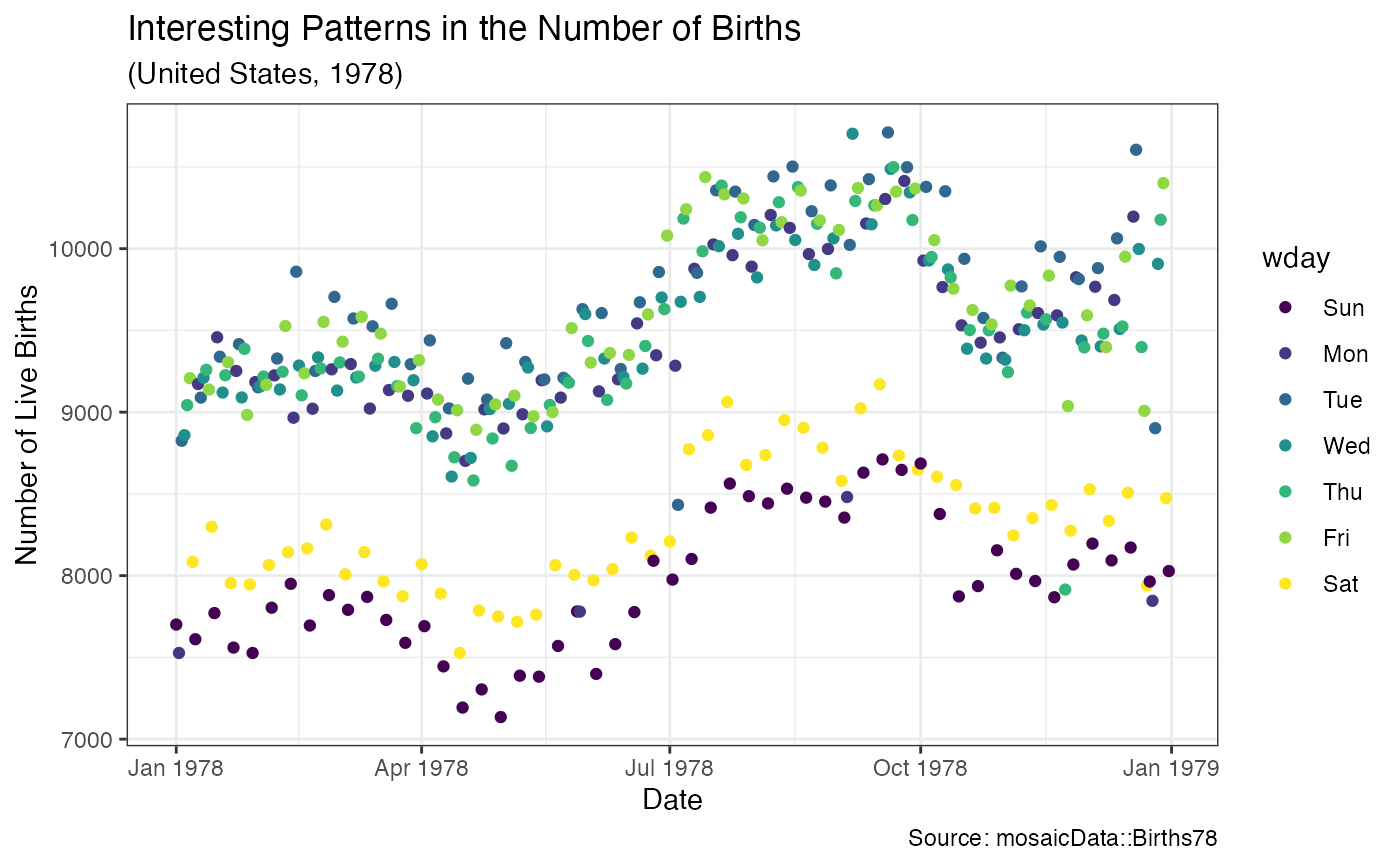Scatterplots in ggformula.

gf_point(
object = NULL,
gformula = NULL,
data = NULL,
...,
alpha,
color,
size,
shape,
fill,
group,
stroke,
xlab,
ylab,
title,
subtitle,
caption,
geom = "point",
stat = "identity",
position = "identity",
show.legend = NA,
show.help = NULL,
inherit = TRUE,
environment = parent.frame()
)

## Arguments

object When chaining, this holds an object produced in the earlier portions of the chain. Most users can safely ignore this argument. See details and examples. A formula with shape y ~ x. Faceting can be achieved by including | in the formula. A data frame with the variables to be plotted. Additional arguments. Typically these are (a) ggplot2 aesthetics to be set with attribute = value, (b) ggplot2 aesthetics to be mapped with attribute = ~ expression, (c) attributes of the layer as a whole, which are set with attribute = value, or (d) arguments for the geom, stat, or position function. Opacity (0 = invisible, 1 = opaque). A color or a formula used for mapping color. A numeric size or a formula used for mapping size. An integer or letter shape or a formula used for mapping shape. A color for filling, or a formula used for mapping fill. Used for grouping. A numeric size of the border or a formula used to map stroke. Label for x-axis. See also gf_labs(). Label for y-axis. See also gf_labs(). Title, sub-title, and caption for the plot. See also gf_labs(). A character string naming the geom used to make the layer. A character string naming the stat used to make the layer. Either a character string naming the position function used for the layer or a position object returned from a call to a position function. A logical indicating whether this layer should be included in the legends. NA, the default, includes layer in the legends if any of the attributes of the layer are mapped. If TRUE, display some minimal help. A logical indicating whether default attributes are inherited. An environment in which to look for variables not found in data.

a gg object

## Specifying plot attributes

Positional attributes (a.k.a, aesthetics) are specified using the formula in gformula. Setting and mapping of additional attributes can be done through the use of additional arguments. Attributes can be set can be set using arguments of the form attribute = value or mapped using arguments of the form attribute = ~ expression.

In formulas of the form A | B, B will be used to form facets using facet_wrap() or facet_grid(). This provides an alternative to gf_facet_wrap() and gf_facet_grid() that is terser and may feel more familiar to users of lattice.

## Evaluation

Evaluation of the ggplot2 code occurs in the environment of gformula. This will typically do the right thing when formulas are created on the fly, but might not be the right thing if formulas created in one environment are used to create plots in another.

ggplot2::geom_point(), gf_line(), gf_jitter()

## Examples

gf_point()
#> gf_point() uses
#>     * a formula with shape y ~ x.
#>     * geom:  point
#>     * key attributes:  alpha, color, size, shape, fill, group, stroke
#>
#> For more information, try ?gf_pointgf_point((10 * ((1:25) %/% 10)) ~ ((1:25) %% 10),
shape = 1:25,
fill = "skyblue", color = "navy", size = 4, stroke = 1, data = NA
)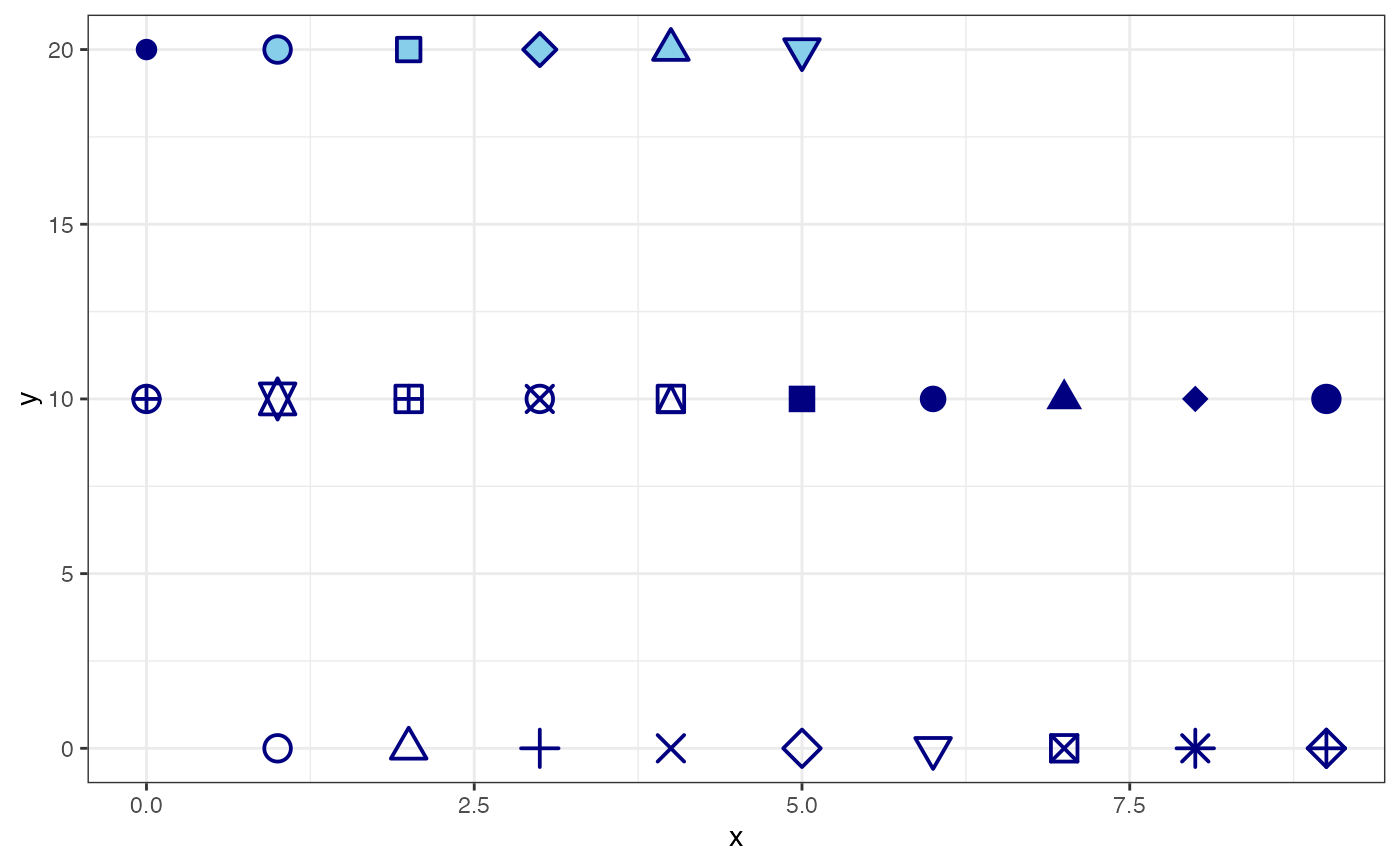gf_point(mpg ~ hp, color = ~cyl, size = ~wt, data = mtcars)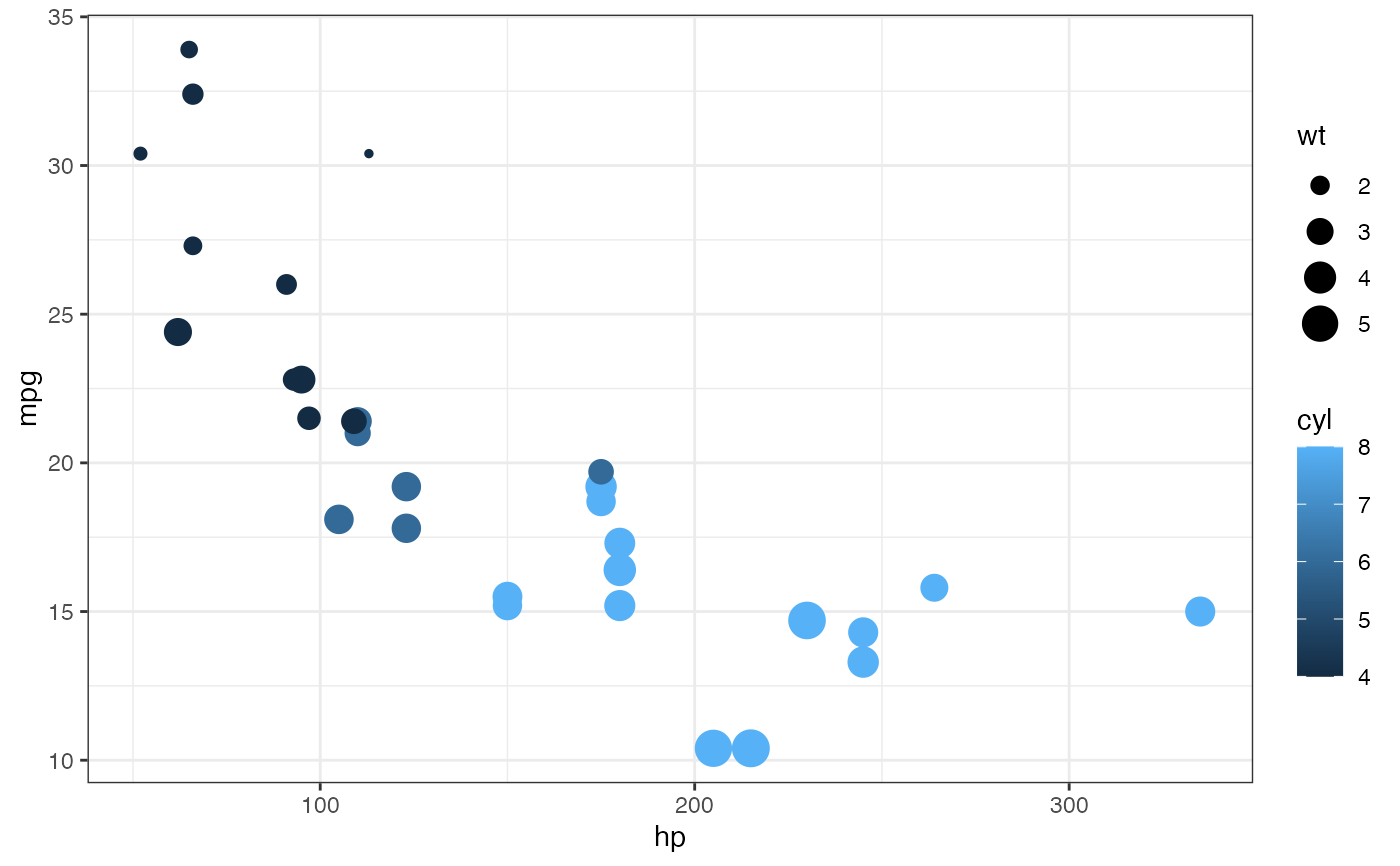# faceting -- two ways
gf_point(mpg ~ hp, data = mtcars) %>%
gf_facet_wrap(~am)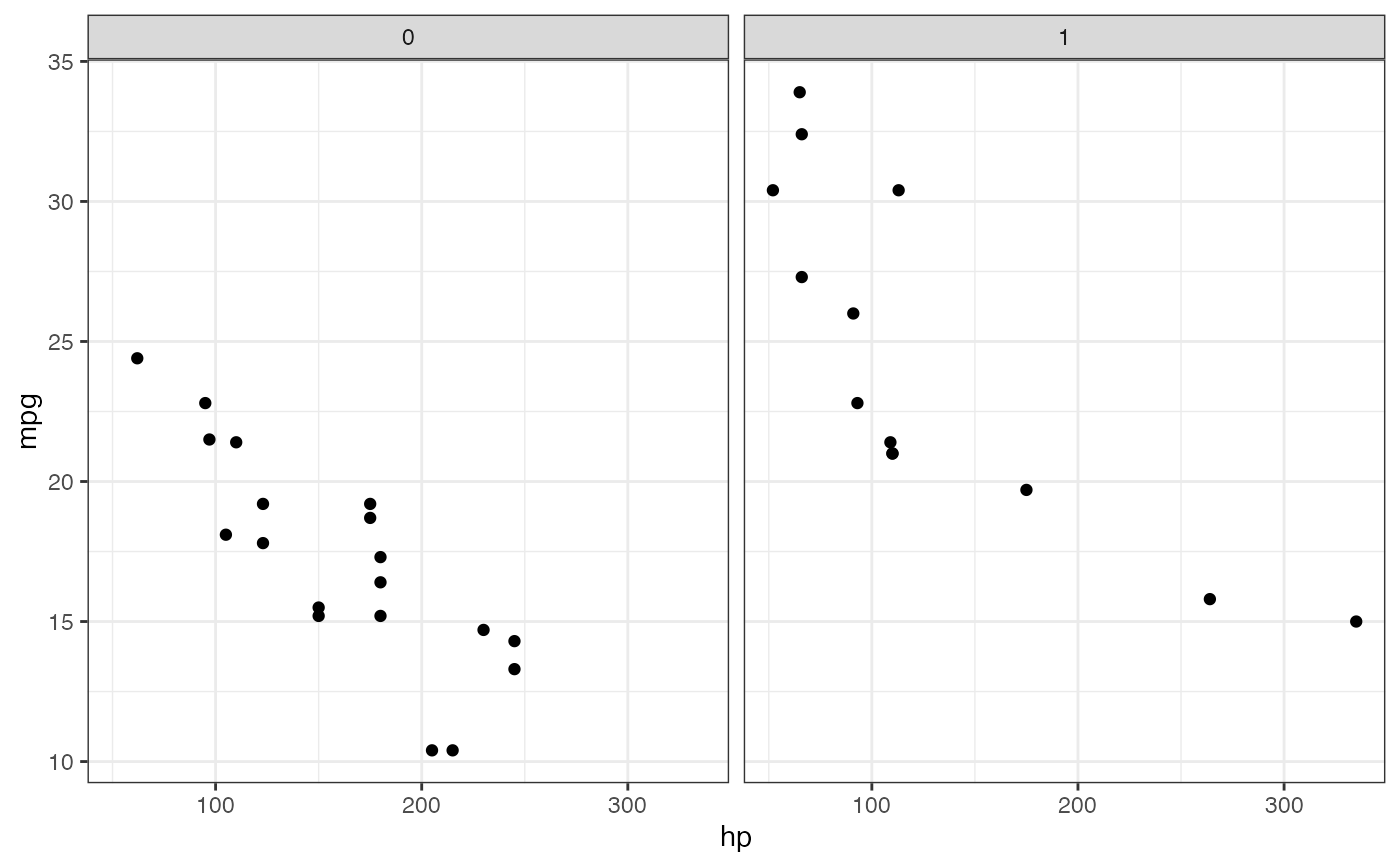gf_point(mpg ~ hp | am, group = ~cyl, data = mtcars)gf_point(mpg ~ hp | ~am, group = ~cyl, data = mtcars)gf_point(mpg ~ hp | am ~ ., group = ~cyl, data = mtcars)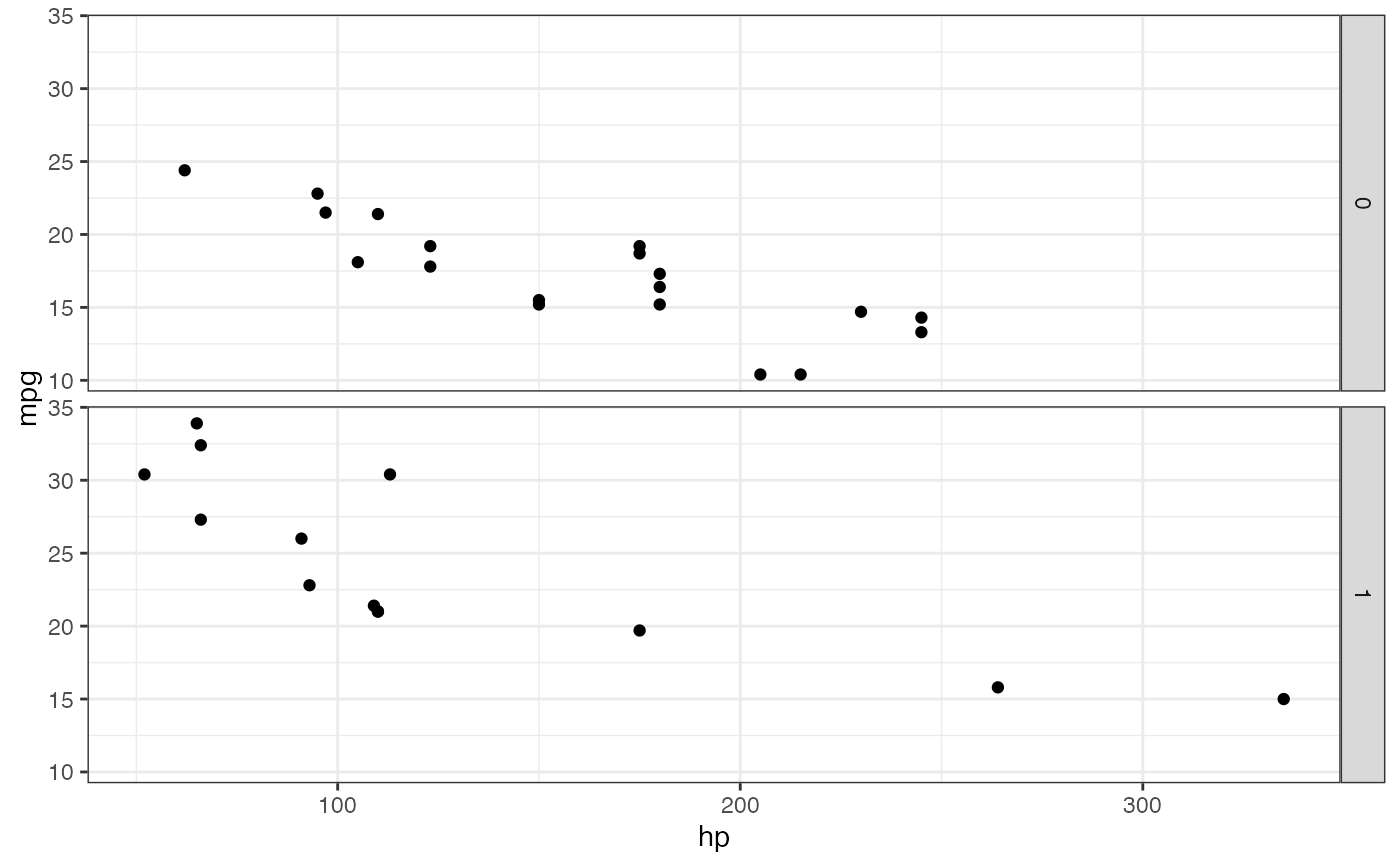# Chaining in the data
mtcars %>% gf_point(mpg ~ wt)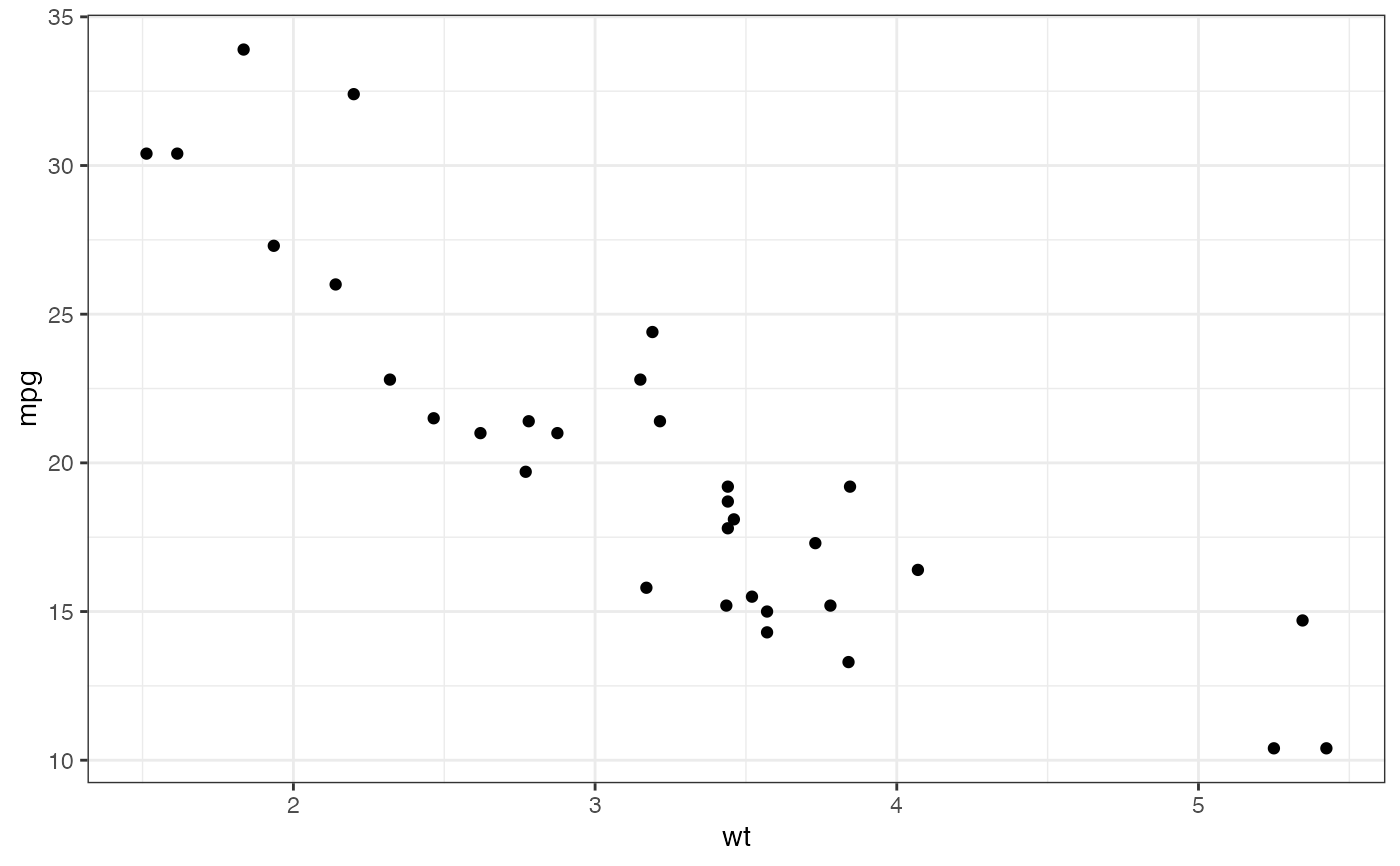# short cuts for main labels in the plot
gf_point(births ~ date,
color = ~wday, data = mosaicData::Births78,
xlab = "Date", ylab = "Number of Live Births",
title = "Interesting Patterns in the Number of Births",
subtitle = "(United States, 1978)",
caption = "Source: mosaicData::Births78"
)